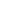# All India Multispecialty Hospitals Ranking Survey 2016

A robust approach was followed to arrive at the final rankings. The step by step process is given below: Factual Score The information collected corresponding to similar parameters as in the perceptual survey A detailed scoring system has been developed for each parameter After assigning scores to each parameter, raw scores were calculated The raw scores were weighted by their importance to arrive at weighted scores The final entity score were calculated as in the perception survey Perceptual Score Ratings on various parameters for each entities Calculating importance of various parameters to arrive at weights for each parameter ­ we use a regression model to arrive at the importance of various parameters Calculation of raw scores and weighted scores for each parameter Calculation of overall score for each hospitals using importance weightage The final score for a hospital were a weighted average of respective hospitals Perception Score and Factual Score equal weightage given to both…
Formore Details : http://epaperbeta.timesofindia.com/Article.aspx?eid=31809&articlexml=Research-Methodology-29042016452058*
*
*
*